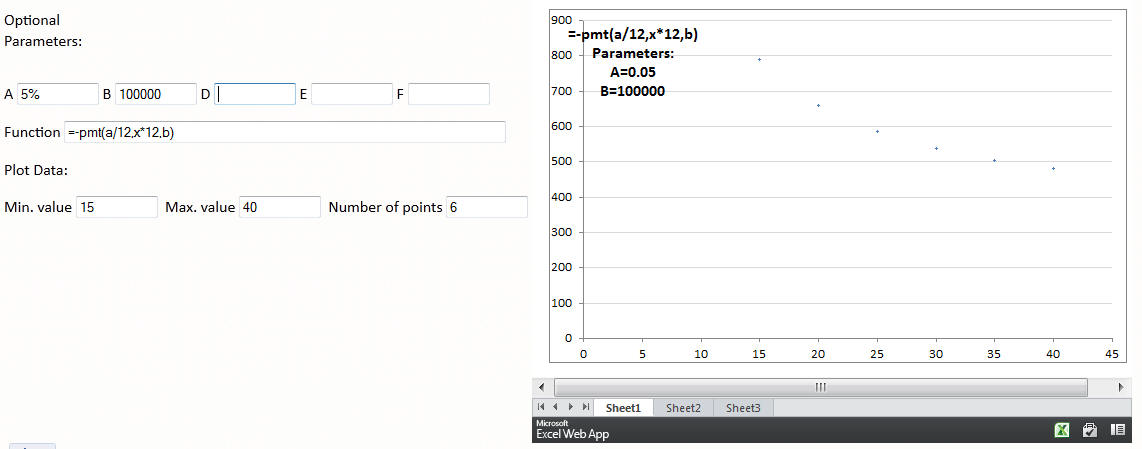﻿ Excel Mashup
You are on the Home > Books and Journal Articles > Data Visualization with Microsoft Excel > Excel on the web - Excel MashupShare Your

# Graph any Excel formula

Interactively graph any Excel formula as a function of a single variable.

Update the various fields in the form below and simply TAB or ENTER to update the graph.  As an example, to see how the monthly payment for a mortgage would vary with the duration of the mortgage, do the following: designate A as the annual interest rate and B as the principal.  So, enter, say 3% in A and 100,000 in B.  Then, in the formula field enter =-pmt(A/12,X*12,B).  Finally, set the min. value to 15, the max. value to 40, and the number of points to, say, 200.  For more see Monthly mortgage payment as a function of the loan duration.

After changing a field value use TAB or ENTER to update the graph.

Optional Formula Parameters:
Parameters are optional variables used in the formula, as in the default example.

Formula:
Enter any legitimate Excel formula.  For the independent variable (the values on the horizontal axis) use the literal X, as in the default example.

Plot Parameters:
Specify the minimum and maximum values for the X variable.
The maximum number of data points currently supported is 1,000.

Min. value
Max. value
Number of points

The content of a field can be either a number or a valid Excel formula.  So, to always plot the default example's normal distribution from mean - 3 * sigma to mean + 3 * sigma, enter in the Min. value field =A-3*B and in the Max. value field =A+3*B.  Now, any changes in the mean (parameter A) or the standard deviation (parameter B) will also automatically adjust the minimum and maximum X values shown.

On occasion, there might be an "inactivity timeout" server error.  If that happens, click the Update Chart button.

For the curious reader, this webpage uses the new "Excel mashup" capability to integrate the information on the web page with the information in the Excel workbook.

# Examples

To plot a standard normal distribution, the formula would be =NORM.S.DIST(X,FALSE).

The formula can include up to 5 optional parameters, named A, B, D, E, and F.  So, to plot a normal distribution with the mean given by A and the standard deviation given by B, one would use =NORM.DIST(X,A,B,FALSE).  Now, enter the desired mean in the field A and the standard deviation in the field B.

After entering a value in any field TAB or ENTER  to update the chart.

## Monthly mortgage payment as a function of the loan duration

To see how the monthly payment for a mortgage would vary with the duration of the mortgage, do the following: designate A as the annual interest rate and B as the principal.  Then, use the formula =-pmt(A/12,X*12,B).  Specify the minimum X value (the number of years) as 15, the maximum x value as 40, and ask for 200 data points.Of course, the typical mortgage is available for only certain durations such as 15 years, 30 years, or 40 years.  So, to see the discrete points, reduce the number of data points to, say, 6.  This will yield x values from 15 to 40 spaced 5 years apart.  The points are not very dark (because the default marker size is 2 pt) but one can see the monthly payments at each of the specific loan durations.